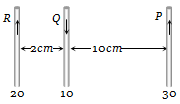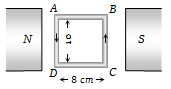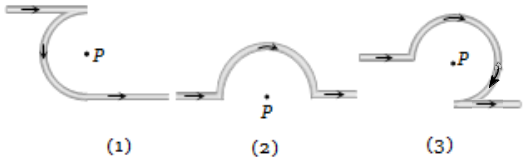Three long, straight and parallel wires carrying currents are arranged as shown in figure. The force experienced by 10 cm length of wire Q is(a) $1.4×{10}^{-4}N$ towards the right

(b) $1.4×{10}^{-4}N$ towards the left

(c) $2.6×{10}^{-4}N$ to the right

(d) $2.6×{10}^{-4}N$ to the left

Concept Questions :-

Force between current carrying wires
High Yielding Test Series + Question Bank - NEET 2020

Difficulty Level:

A 100 turns coil shown in figure carries a current of 2 amp in a magnetic field $B=0.2Wb/{m}^{2}$. The torque acting on the coil is(a) 0.32 Nm tending to rotate the side AD out of the page

(b) 0.32 Nm tending to rotate the side AD into the page

(c) 0.0032 Nm tending to rotate the side AD out of the page

(d) 0.0032 Nm tending to rotate the side AD into the page

Concept Questions :-

Magnetic moment
High Yielding Test Series + Question Bank - NEET 2020

Difficulty Level:

A current of 5 amperes is flowing in a wire of length 1.5 meters. A force of 7.5 N acts on it when it is placed in a uniform magnetic field of 2 Tesla. The angle between the magnetic field and the direction of the current is:

1. 30$°$                     2. 45°

3. 60°                     4. 90°

Concept Questions :-

Lorentz force
High Yielding Test Series + Question Bank - NEET 2020

Difficulty Level:

The field normal to the plane of a wire of n turns and radius r which carries a current i is measured on the axis of the coil at a small distance h from the centre of the coil. This is smaller than the field at the centre by the fraction

(a) $\frac{3}{2}\frac{{\mathrm{h}}^{2}}{{\mathrm{r}}^{2}}$                       (b) $\frac{2}{3}\frac{{\mathrm{h}}^{2}}{{\mathrm{r}}^{2}}$

(c)  $\frac{3}{2}\frac{{\mathrm{r}}^{2}}{{\mathrm{h}}^{2}}$                       (d) $\frac{2}{3}\frac{{\mathrm{r}}^{2}}{{\mathrm{h}}^{2}}$

Concept Questions :-

Magnetic field due to various cases
High Yielding Test Series + Question Bank - NEET 2020

Difficulty Level:

In hydrogen atom, the electron is making $6.6×{10}^{15}rev/sec$ around the nucleus in an orbit of radius 0.528 Å. The magnetic moment $\left(A-{m}^{2}\right)$ will be

(a) $1×{10}^{-15}$                      (b) $1×{10}^{-10}$

(c) $1×{10}^{-23}$                       (d) $1×{10}^{-27}$

Concept Questions :-

Magnetic moment
High Yielding Test Series + Question Bank - NEET 2020

Difficulty Level:

A proton of mass $1.67×{10}^{-27}kg$ and charge $1.6×{10}^{-19}C$ is projected with a speed of $2×{10}^{6}m/s$ at an angle of $60°$ to the X-axis. If a uniform magnetic field of 0.104 Tesla is applied along Y-axis, the path of the proton is:

1. A circle of radius = 0.2 m and time period $\mathrm{\pi }×{10}^{-7}\mathrm{s}$

2. A circle of radius = 0.1 m and time period $2\mathrm{\pi }×{10}^{-7}\mathrm{s}$

3. A helix of radius = 0.1 m and time period $2\mathrm{\pi }×{10}^{-7}\mathrm{s}$

4. A helix of radius = 0.2 m and time period $4\mathrm{\pi }×{10}^{-7}\mathrm{s}$

Concept Questions :-

Lorentz force
High Yielding Test Series + Question Bank - NEET 2020

Difficulty Level:

The magnetic field at the centre of a circular coil of radius r is $\mathrm{\pi }$ times that due to a long straight wire at a distance r from it, for equal currents. Figure here shows three cases : in all cases the circular part has radius r and straight ones are infinitely long. For same current the B field at the centre P in cases 1, 2, 3 have the ratio(a)

(b) $\left(-\frac{\mathrm{\pi }}{2}+1\right)\mathbf{:}\left(\frac{\mathrm{\pi }}{2}+1\right)\mathbf{:}\left(\frac{3\mathrm{\pi }}{4}+\frac{1}{2}\right)$

(c) $-\frac{\mathrm{\pi }}{2}\mathbf{:}\frac{\mathrm{\pi }}{2}\mathbf{:}3\frac{\mathrm{\pi }}{4}$

(d) $\left(-\frac{\mathrm{\pi }}{2}-1\right)\mathbf{:}\left(\frac{\mathrm{\pi }}{2}-\frac{1}{4}\right)\mathbf{:}\left(\frac{3\mathrm{\pi }}{4}+\frac{1}{2}\right)$

Concept Questions :-

Magnetic field due to various cases
High Yielding Test Series + Question Bank - NEET 2020

Difficulty Level:

A proton and a deutron both having the same kinetic energy, enter perpendicularly into a uniform magnetic field B. For motion of proton and deutron on circular path of radius ${R}_{p}$and ${R}_{d}$ respectively, the correct statement is

(a) ${R}_{d}=\sqrt{2}{R}_{p}$            (b) ${R}_{p}={R}_{p}/\sqrt{2}$

(c) ${R}_{d}={R}_{p}$                  (d) ${R}_{d}=2{R}_{p}$

Concept Questions :-

Lorentz force
High Yielding Test Series + Question Bank - NEET 2020

Difficulty Level:

Two straight long conductors AOB and COD are perpendicular to each other and carry currents ${\mathrm{i}}_{1}$ and ${\mathrm{i}}_{2}$ . The magnitude of the magnetic induction at a point P at a distance a from the point O in a direction perpendicular to the plane ACBD is:

1. $\frac{{\mathrm{\mu }}_{0}}{2\mathrm{\pi a}}\left({\mathrm{i}}_{1}+{\mathrm{i}}_{2}\right)$                    2. $\frac{{\mathrm{\mu }}_{0}}{2\mathrm{\pi a}}\left({\mathrm{i}}_{1}-{\mathrm{i}}_{2}\right)$

3. $\frac{{\mathrm{\mu }}_{0}}{2\mathrm{\pi a}}{\left({\mathrm{i}}_{1}^{2}+{\mathrm{i}}_{2}^{2}\right)}^{1/2}$                4. $\frac{{\mathrm{\mu }}_{0}}{2\mathrm{\pi a}}\frac{{\mathrm{i}}_{1}{\mathrm{i}}_{2}}{\left({\mathrm{i}}_{1}+{\mathrm{i}}_{2}\right)}$

Concept Questions :-

Magnetic field due to various cases
High Yielding Test Series + Question Bank - NEET 2020

Difficulty Level:

The charge on a particle Y is double the charge on particle X. These two particles X and Y after being accelerated through the same potential difference enter a region of the uniform magnetic field and describe circular paths of radii ${R}_{1}$ and ${R}_{2}$ respectively. The ratio of the mass of X to that of Y is:

(a) ${\left(\frac{2{R}_{1}}{{R}_{2}}\right)}^{2}$                      (b) ${\left(\frac{{R}_{1}}{2{R}_{2}}\right)}^{2}$

(c)  $\frac{{R}_{1}^{2}}{2{R}_{2}^{2}}$                           (d) $\frac{2{R}_{1}}{{R}_{2}}$

Concept Questions :-

Lorentz force